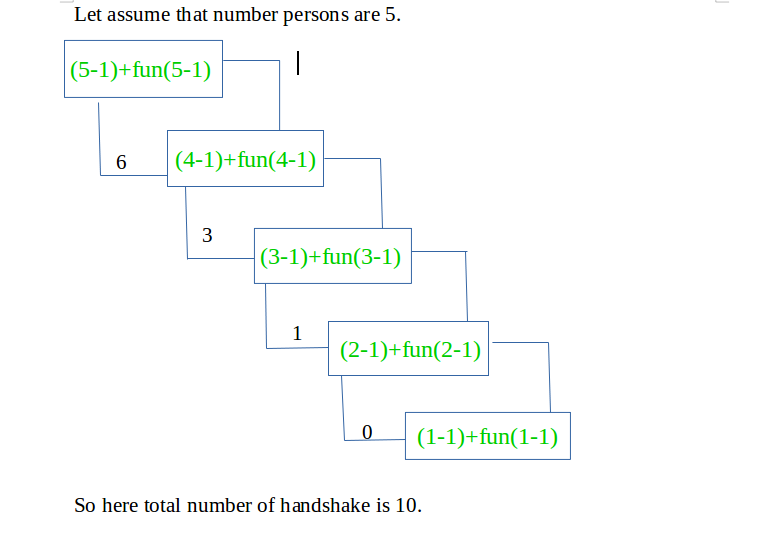# Number of handshakes such that a person shakes hands only once

• Difficulty Level : Easy
• Last Updated : 02 May, 2022

There is N number of people at a party. Find the total number of handshakes such that a person can handshake only once.

Examples:

```Input : 5
Output : 10

Input : 9
Output : 36 ```

We can see a recursive nature in the problem.

```// n-th person has (n-1) choices and after
// n-th person chooses a person, problem
// recurs for n-1.
handshake(n) = (n-1) + handshake(n-1)

// Base case
handshake(0) = 0 ```Below is the implementation of the above recursive formula.

## C++

 `// Recursive C++ program to count total number of handshakes``// when a person can shake hand with only one.``#include ``using` `namespace` `std;` `// Function to find all possible handshakes``int` `handshake(``int` `n)``{` `    ``// When n becomes 0 that means all the persons have done``    ``// handshake with other``    ``if` `(n == 0)``        ``return` `0;``    ``else``        ``return` `(n - 1) + handshake(n - 1);``}` `// Driver code``int` `main()``{``    ``int` `n = 9;``    ``cout << ``" "` `<< handshake(n);``    ``return` `0;``}` `// This code is contributed by Aditya Kumar (adityakumar129)`

## C

 `// Recursive C program to count total number of  handshakes``// when a person can shake hand with only one.``#include ` `// function to find all possible handshakes``int` `handshake(``int` `n)``{``    ``// when n becomes 0 that means all the persons have done``    ``// handshake with other``    ``if` `(n == 0)``        ``return` `0;``    ``else``        ``return` `(n - 1) + handshake(n - 1);``}` `int` `main()``{``    ``int` `n = 9;``    ``printf``(``"%d"``, handshake(n));``    ``return` `0;``}` `// This code is contributed by Aditya Kumar (adityakumar129)`

## Java

 `// Recursive Java program to count total number of``// handshakes when a person can shake hand with only one.``import` `java.io.*;` `class` `GFG {` `    ``// function to find all possible handshakes``    ``static` `int` `handshake(``int` `n)``    ``{``        ``// when n becomes 0 that means all the persons have``        ``// done handshake with other``        ``if` `(n == ``0``)``            ``return` `0``;``        ``else``            ``return` `(n - ``1``) + handshake(n - ``1``);``    ``}` `    ``// Driver Code``    ``public` `static` `void` `main(String[] args)``    ``{``        ``int` `n = ``9``;``        ``System.out.print(handshake(n));``    ``}``}` `// This code is contributed by Aditya Kumar (adityakumar129)`

## Python3

 `# Recursive Python program``# to count total number of``# handshakes when a person``# can shake hand with only one.` `# function to find all``# possible handshakes``def` `handshake(n):` `    ``# when n becomes 0 that means``    ``# all the persons have done``    ``# handshake with other``    ``if` `(n ``=``=` `0``):``        ``return` `0``    ``else``:``        ``return` `(n ``-` `1``) ``+` `handshake(n ``-` `1``)` `# Driver Code``n ``=` `9``print``(handshake(n))` `# This code is contributed``# by Shivi_Aggarwal`

## C#

 `// Recursive C# program to``// count total number of``// handshakes when a person``// can shake hand with only one.``using` `System;` `class` `GFG``{` `// function to find all``// possible handshakes``static` `int` `handshake(``int` `n)``{``    ``// when n becomes 0 that``    ``// means all the persons``    ``// have done handshake``    ``// with other``    ``if` `(n == 0)``        ``return` `0;``    ``else``        ``return` `(n - 1) + handshake(n - 1);``}` `// Driver Code``public` `static` `void` `Main (String []args)``{``    ``int` `n = 9;``    ``Console.WriteLine(handshake(n));``}``}` `// This code is contributed``// by Arnab Kundu`

## PHP

 ``

## Javascript

 ``

Output:

`36`

We can come up with a direct formula by expanding the recursion.

```handshake(n) = (n-1) + handshake(n-1)
= (n-1) + (n-2) + handshake(n-2)
= (n-1) + (n-2) + .... 1 + 0
= n * (n - 1)/2  ```

## C++

 `// Recursive CPP program to count total number of handshakes``// when a person can shake hand with only one.``#include ``using` `namespace` `std;` `// function to find all possible handshakes``int` `handshake(``int` `n) { ``return` `n * (n - 1) / 2; }` `int` `main()``{``    ``int` `n = 9;``    ``cout << handshake(n) << endl;``    ``return` `0;``}` `// This code is contributed by Aditya Kumar (adityakumar129)`

## C

 `// Recursive CPP program to count total number of handshakes``// when a person can shake hand with only one.``#include ` `// function to find all possible handshakes``int` `handshake(``int` `n) { ``return` `n * (n - 1) / 2; }` `int` `main()``{``    ``int` `n = 9;``    ``printf``(``"%d"``, handshake(n));``    ``return` `0;``}` `// This code is contributed by Aditya Kumar (adityakumar129)`

## Java

 `// Recursive Java program to count total number of``// handshakes when a person can shake hand with only one.``class` `GFG {` `    ``// function to find all possible handshakes``    ``static` `int` `handshake(``int` `n) { ``return` `n * (n - ``1``) / ``2``; }` `    ``// Driver code``    ``public` `static` `void` `main(String args[])``    ``{``        ``int` `n = ``9``;``        ``System.out.println(handshake(n));``    ``}``}` `// This code is contributed by Aditya Kumar (adityakumar129)`

## Python3

 `# Recursive Python program``# to count total number of``# handshakes when a person``# can shake hand with only one.` `# function to find all``# possible handshakes``def` `handshake(n):``    ` `    ``return` `int``(n ``*` `(n ``-` `1``) ``/` `2``)` `# Driver Code``n ``=` `9``print``(handshake(n))``    ` `# This code is contributed``# by Shivi_Aggarwal`

## C#

 `// Recursive C# program to``// count total number of``// handshakes when a person``// can shake hand with only one.``using` `System;` `class` `GFG``{``    ` `// function to find all``// possible handshakes``static` `int` `handshake(``int` `n)``{``    ``return` `n * (n - 1) / 2;``}` `// Driver code``static` `public` `void` `Main ()``{``    ``int` `n = 9;``    ``Console.WriteLine(handshake(n));``}``}` `// This code is contributed by Sachin`

## PHP

 ``

## Javascript

 ``

Output:

`36`

My Personal Notes arrow_drop_up